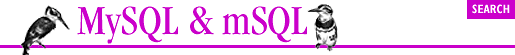home | O'Reilly's CD bookshelfs | FreeBSD | Linux | Cisco | Cisco Exam## 10.2. An Example DBI Application

To begin with we are interested in creating tests for the various subjects. To do this we need a table that contains names and ID numbers for the tests. We also need a separate table for each test. This table will contain the scores for all of the students as well as a perfect score for comparison. The test table has the following structure:

```CREATE TABLE test (
id INT NOT NULL AUTO_INCREMENT,
name CHAR(100),
subject INT,
num INT
)```

The individual tests have table structures like this:

```CREATE TABLE t7 (
id INT NOT NULL,
q1 INT,
q2 INT,
q3 INT,
q4 INT,
total INT
)```

The table name is t followed by the test ID number from the test table. The user determines the number of questions when he or she creates the table. The total field is the sum of all of the questions.

This example is a MySQL example. Of course, the API is identical for mSQL. The only "glitch" is with sequence generation. Remember that where MySQL automatically generates the next ID for the test table because of the AUTO_INCREMENT keyword, mSQL expects you to create a sequence on the test table and SELECT the _seq value before doing your insert.

```#!/usr/bin/perl -w

use strict;
require my_end;

use CGI qw(:standard);
my \$output = new CGI;
use_named_parameters(1);

# Use the DBI module.
use DBI;
# DBI::connect() uses the format 'DBI:driver:database', in our case we are
# using the MySQL driver and accessing the 'teach' database.
my \$dbh = DBI->connect('DBI:mysql:teach');
# The add action itself is broken up into three separate functions. The first # function, add, prints out the template form for the user to create a new # # test.
\$subject = param('subject') if (param('subjects'));
\$subject = "" if \$subject eq 'all';

print header, start_html('title'=>'Create a New Test',
'BGCOLOR'=>'white');
print <<END_OF_HTML;
<H1>Create a New Test</h1>
<FORM ACTION="test.cgi" METHOD=POST>
Subject:
END_OF_HTML
my @ids = ();
my %subjects = ();
my \$out2 = \$dbh->prepare("select id,name from subject order by name");
\$out2->execute;
# DBI::fetchrow_array() is exactly analogous to Msql::fetchrow()
while(my(\$id,\$subject)=\$out2->fetchrow_array) {
push(@ids,\$id);
\$subjects{"\$id"} = \$subject;
}
'values'=>[@ids],
'default'=>\$subject,
'labels'=>\%subjects);
print <<END_OF_HTML;
<br>
Number of Questions: <INPUT NAME="num" SIZE=5><br>
A name other identifier (such as a date) for the test:
<INPUT NAME="name" SIZE=20>
<p>
<INPUT TYPE=SUBMIT VALUE=" Next Page ">
<INPUT TYPE=RESET>
</form></body></html>
END_OF_HTML
}```

This function displays a form allowing the user to choose a subject for the test along with the number of questions and a name. In order to print out a list of available subjects, the table of subjects is queried. When using a SELECT query with DBI, the query must first be prepared and then executed. The DBI::prepare function is useful with certain database servers which allow you to perform operations on prepared queries before executing them. With MySQL and mSQL however, it simply stores the query until the DBI::execute function is called.

The output of this function is sent to the add2 function as shown in the following:

```sub add2 {
my \$subject = param('subjects');
my \$num = param('num');
\$name = param('name') if param('name');

my \$out = \$dbh->prepare("select name from subject where id=\$subject");
\$out->execute;
my (\$subname) = \$out->fetchrow_array;

print header, start_html('title'=>"Creating test for \$subname",
'BGCOLOR'=>'white');
print <<END_OF_HTML;
<H1>Creating test for \$subname</h1>
<h2>\$name</h2>
<p>
<FORM ACTION="test.cgi" METHOD=POST>
<INPUT TYPE=HIDDEN NAME="subjects" VALUE="\$subject">
<INPUT TYPE=HIDDEN NAME="num" VALUE="\$num">
<INPUT TYPE=HIDDEN NAME="name" VALUE="\$name">
Enter the point value for each of the questions. The points need not
<p>
END_OF_HTML
for (1..\$num) {
print qq%\$_: <INPUT NAME="q\$_" SIZE=3> %;
if (not \$_ % 5) { print "<br>\n"; }
}
print <<END_OF_HTML;
<p>
Enter the text of the test:<br>
<TEXTAREA NAME="test" ROWS=20 COLS=60>
</textarea>
<p>
<INPUT TYPE=SUBMIT VALUE="Enter Test">
<INPUT TYPE=RESET>
</form></body></html>
END_OF_HTML
}```

In this function, a form for the test is dynamically generated based on the parameters entered in the last form. The user can enter the point value for each question on the test and the full text of the test as well. The output of this function is then sent to the final function, add3, as shown in the following:

```sub add3 {
my \$subject = param('subjects');
my \$num = param('num');

\$name = param('name') if param('name');

my \$qname;
(\$qname = \$name) =~ s/'/\\'/g;
my \$q1 = "insert into test (id, name, subject, num) values (
'', '\$qname', \$subject, \$num)";

my \$in = \$dbh->prepare(\$q1);
\$in->execute;

# Retrieve the ID value MySQL created for us
my \$id = \$in->insertid;

my \$query = "create table t\$id (
id INT NOT NULL,
";

my \$def = "insert into t\$id values ( 0, ";

my \$total = 0;
my @qs = grep(/^q\d+\$/,param);
foreach (@qs) {
\$query .= \$_ . " INT,\n";
my \$value = 0;
\$value = param(\$_) if param(\$_);
\$def .= "\$value, ";
\$total += \$value;
}
\$query .= "total INT\n)";
\$def .= "\$total)";

my \$in2 = \$dbh->prepare(\$query);
\$in2->execute;
my \$in3 = \$dbh->prepare(\$def);
\$in3->execute;

# Note that we store the tests in separate files. This is
# useful when dealing with mSQL because of its lack of BLOBs.
# (The TEXT type provided with mSQL 2 would work, but
# inefficently.)
# Since we are using MySQL, we could just as well
# stick the entire test into a BLOB.
open(TEST,">teach/tests/\$id") or die("A: \$id \$!");
print TEST param('test'), "\n";
close TEST;

'BGCOLOR'=>'white');
print <<END_OF_HTML;
<H1>Test Created</h1>
<p>
The test has been created.
<p>